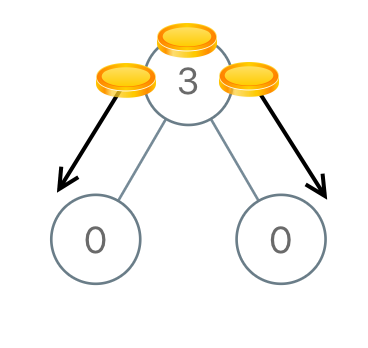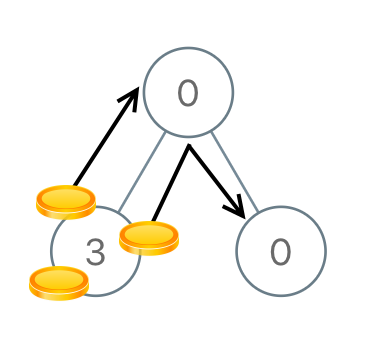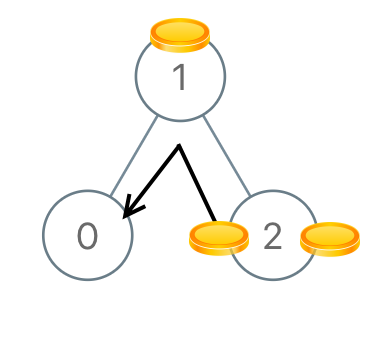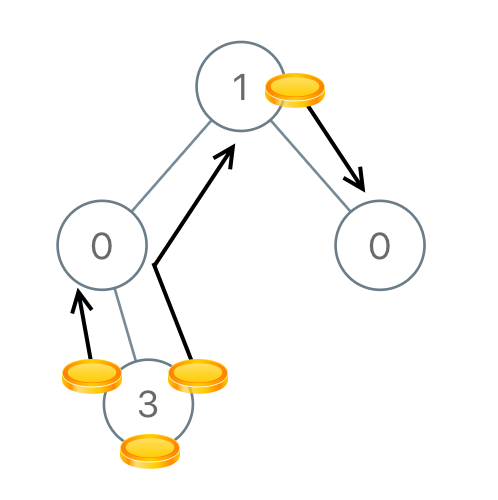979. Distribute Coins in Binary Tree

You are given the `root` of a binary tree with `n` nodes where each `node` in the tree has `node.val` coins and there are `n` coins total.

In one move, we may choose two adjacent nodes and move one coin from one node to another. (A move may be from parent to child, or from child to parent.)

Return the number of moves required to make every node have exactly one coin.

Example 1:```Input: root = [3,0,0]
Output: 2
Explanation: From the root of the tree, we move one coin to its left child, and one coin to its right child.
```

Example 2:```Input: root = [0,3,0]
Output: 3
Explanation: From the left child of the root, we move two coins to the root [taking two moves].  Then, we move one coin from the root of the tree to the right child.
```

Example 3:```Input: root = [1,0,2]
Output: 2
```

Example 4:```Input: root = [1,0,0,null,3]
Output: 4
```

Constraints:

• The number of nodes in the tree is `n`.
• `1 <= n <= 100`
• `0 <= Node.val <= n`
• The sum of `Node.val` is `n`.

979. Distribute Coins in Binary Tree
``````struct Solution;
use rustgym_util::*;

trait Postorder {
fn postorder(&self, sum: &mut i32) -> i32;
}

impl Postorder for TreeLink {
fn postorder(&self, sum: &mut i32) -> i32 {
if let Some(node) = self {
let val = node.borrow().val;
let left = &node.borrow().left;
let right = &node.borrow().right;
let l = left.postorder(sum);
let r = right.postorder(sum);
let m = val + l + r - 1;
*sum += m.abs();
m
} else {
0
}
}
}

impl Solution {
fn distribute_coins(root: TreeLink) -> i32 {
let mut res = 0;
root.postorder(&mut res);
res
}
}

#[test]
fn test() {
let root = tree!(3, tree!(0), tree!(0));
let res = 2;
assert_eq!(Solution::distribute_coins(root), res);
let root = tree!(0, tree!(3), tree!(0));
let res = 3;
assert_eq!(Solution::distribute_coins(root), res);
let root = tree!(1, tree!(0), tree!(2));
let res = 2;
assert_eq!(Solution::distribute_coins(root), res);
let root = tree!(1, tree!(0, None, tree!(3)), tree!(0));
let res = 4;
assert_eq!(Solution::distribute_coins(root), res);
}
``````